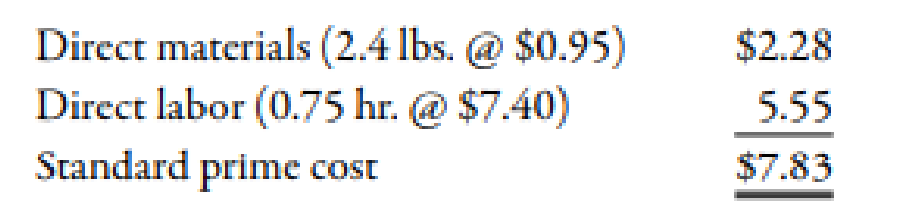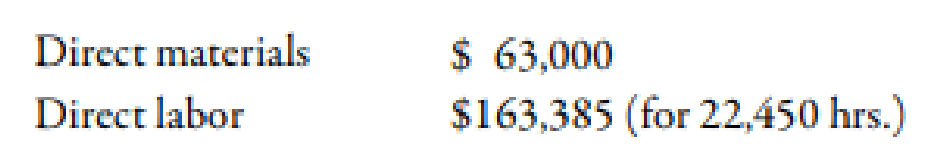Chapter 10, Problem 52E### Managerial Accounting: The Corners...

7th Edition
Maryanne M. Mowen + 2 others
ISBN: 9781337115773

#### Solutions

Chapter
Section### Managerial Accounting: The Corners...

7th Edition
Maryanne M. Mowen + 2 others
ISBN: 9781337115773
Textbook Problem
380 views

# 10-52 Materials and Labor VariancesRefer to the information for Deporte Company above.Required:Compute the materials and labor variances associated with the changeover activity, labeling each variance as favorable or unfavorable.Use the following information for Exercises 10-52 and 10-53:Deporte Company produces single-colored T-shirts. Materials for the shirts are dyed in large vats. After dying the materials for a given color, the vats must be cleaned and prepared for the next batch of materials to be colored. The following standards for changeover for a given batch have been established:During the year, 79,500 pounds of material were purchased and used for the changeover activity. There were 30,000 batches produced, with the following actual prime costs:To determine

Calculate the value of material variance and labor variance. Also, mark each variance as favorable or unfavorable.

Explanation

Variance:

The amount obtained when actual cost is deducted from budgeted cost is known as variance. Variance is calculated to find whether the cost is over applied or under applied.

Use the following formula to calculate material price variance:

Material Price Variance=((Standard Price×Actual Quantity)(Actual Price×Actual Quantity))

Substitute$63,000 for actual price and actual quantity,$0.95 for standard price and 79,500 for actual quantity in the above formula.

Material Price Variance=($0.95×79,500)($63,000)=$12,525(F) Therefore, the material price variance is$12,525(F).

Use the following formula to calculate material usage variance:

Material Usage Variance=((Standard Price×Actual Quantity)(Standard Price×Standard Quantity1))

Substitute $0.95 for standard price, 79,500 for actual quantity and 72,000 for standard quantity in the above formula. Material Usage Variance=($0.95×79,500)($0.95×72,000)=$7,125(F)

Therefore, the material usage variance is $7,125(F). Use the following formula to calculate labor rate variance: Labor Rate Variance=((Standard Rate×Actual Hours)(Actual Rate×Actual Hours)) Substitute$163,385 for actual rate and actual hours, \$7

### Still sussing out bartleby?

Check out a sample textbook solution.

See a sample solution

#### The Solution to Your Study Problems

Bartleby provides explanations to thousands of textbook problems written by our experts, many with advanced degrees!

Get Started